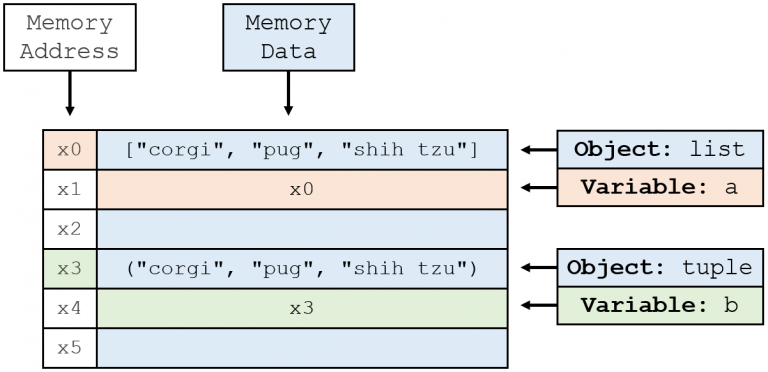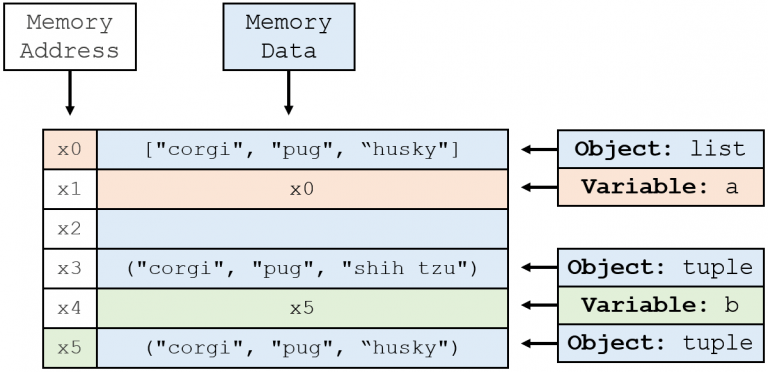# What is the Difference Between List and Tuple in Python?

Published Jan 18, 2020  ∙  Updated May 2, 2022

So you’re learning Python and you’ve come across lists and tuples.

Let’s try to understand what they are and why they exist.

## Similarities between lists and tuples

Both lists and tuples are data types that can store multiple elements.

``````a = ["corgi", "pug", "shih tzu"] # list
b = ("corgi", "pug", "shih tzu") # tuple
``````

They both can hold elements of different types within the same sequence.

``````c = ["corgi", 2, 3.0] # list
d = ("corgi", 2, 3.0) # tuple
``````

And, unlike sets and dictionaries, both of them maintain the order of the sequence, making them indexable.

``````c # 2
d # 2
``````

## Differences between lists and tuples

### Difference 1: Syntax

Lists are created using brackets `[]` while tuples are created using parentheses `()`.

``````a = ["corgi", "pug", "shih tzu"] # list
b = ("corgi", "pug", "shih tzu") # tuple
``````

They can also be created using the `list()` and `tuple()` functions.

``````a = ["corgi", "pug", "shih tzu"] # list
b = tuple(a)
c = list(b)
b # ('corgi', 'pug', 'shih tzu')
c # ['corgi', 'pug', 'shih tzu']
``````

This is a clean way to convert from lists to tuples or from tuples to lists.

### Difference 2: Mutability

Lists are mutable, meaning that a list can be modified after creation.

Tuples are immutable, meaning that a tuple cannot be modified after creation.

Let’s try to modify a list and turn the shih tzu into a husky.

``````a = ["corgi", "pug", "shih tzu"] # list
a = "husky"
a # ['corgi', 'pug', 'husky']
``````

We’ve successfully modified our list!

Let’s see what happens with a tuple.

``````b = ("corgi", "pug", "shih tzu") # tuple
b = "husky"
``````

We get this error:

``````Traceback (most recent call last):
File "<stdin>", line 1, in <module>
TypeError: 'tuple' object does not support item assignment
``````

Ouch. So we can’t turn our shih tzu into a husky using a tuple.

That’s because tuples are immutable and, therefore, cannot be modified. The term “modified” refers to any of the following:

• Removing elements
• Changing elements

Don’t be fooled, though. It may seem like tuples are mutable in the following example:

``````b = ("corgi", "pug", "shih tzu") # tuple
b = ("corgi", "pug", "husky")
b # ('corgi', 'pug', 'husky')
``````

We’ve changed the shih tzu to a husky, so it must be mutable, right?

Nope.

To understand this, we have to first understand how variables and objects work in memory.

## Variables vs. Objects in Memory

Lists and tuples are Python objects that are stored in memory.

`a` and `b` are variables. Variables are pointers to objects. That means that, in memory, `a` and `b` simply hold the address at which the objects are located.

When we initialize list `a` and tuple `b`, the state of memory is as follows:

``````a = ["corgi", "pug", "shih tzu"] # list
b = ("corgi", "pug", "shih tzu") # tuple
``````When we modified the list, which is mutable, with this line:

``````a = "husky"
``````

We were modifying the same list object in the same memory address.

When we run the following line, “modifying” the tuple:

``````b = ("corgi", "pug", "husky")
``````

We are changing the pointer of `b` to another tuple object in memory.

The resulting memory state looks like this:As a result, we can only delete entire tuples or reassign entire tuples. Just know that, in memory, this simply refers to changing pointers and creating completely new Python objects.

So what does this mean for both data types?

## Implications of Mutability

### 1. Available Operations

Because tuples are immutable, they do not have the same number of functions as lists, as seen below.

``````# Available List Operations
a.append(x)
a.clear(iterable)
a.copy()
a.count(x)
a.extend()
a.index(x[, start[, end]])
a.insert(i,x)
a.pop([i])
a.remove(x)
a.reverse()
a.sort()
``````
``````# Available Tuple Operations
b.count(x)
b.index(x[, start[, end]])
``````

### 2. Space Allocation

Because tuples are immutable, their size/space in memory is always constant and smaller than that of a list.

When a list is created, the space in memory is over-allocated in order to make appending new elements faster. We can verify this using the sys.getsizeof() method that will return the size of any given object in bytes.

``````a = ["corgi", "pug", "shih tzu"] # list
b = ("corgi", "pug", "shih tzu") # tuple
import sys
sys.getsizeof(a) # 96
sys.getsizeof(b) # 80
``````

Another really interesting feature of immutability comes when you create two immutable objects that have the same values. We can use the id() function to determine what address in memory at which an object exists. We will test this with strings since they are immutable as well.

``````a = "Difficult"
b = "Simple"
c = "Simple"
id(a) # 140412010628592 = Different
id(b) # 140412010628656 = Same
id(c) # 140412010628656 = Same
``````

As a way to save memory space, Python may combine two different immutable objects into one and simply assign both pointers the same memory address. This is okay because we know that those objects will never change.

This is known as string interning.

The same will hold for any other immutable object such as floats, integers, booleans, etc.

That being said, tuple interning is not quite the same.

While it may be memory efficient to have tuple interning, there is a huge overhead in detecting duplicates for tuples. With a single string or a single integer, it is clear that the entire object is immutable. However, tuples may contain objects that are mutable. Checking that every object is immutable can take a long time if the tuple is large.

Here’s an example:

``````a = (["corgi"], "shih tzu") # list inside a tuple
b = (["corgi"], "shih tzu") # list inside a tuple
b.append("pug")
a # (['corgi'], 'shih tzu')
b # (['corgi', 'pug'], 'shih tzu')
``````

As you can see, the list inside the tuple is still mutable, so it can be changed.

Checking if `a` and `b` refer to the same exact tuple can take a lot of time with a lot of elements.